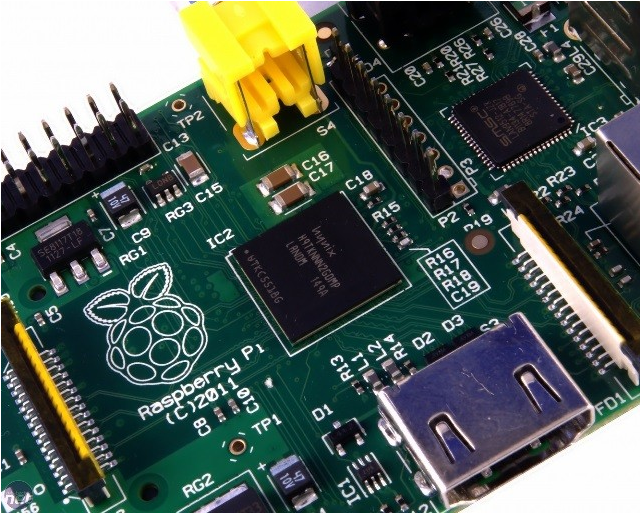# 树莓派存储温度数据并制作交互式图表

Aceryt

0收藏

Raspberry Pi的芯片系统（SoC）具有温度传感器，可用用命令行测量其温度。它可以提供芯片在运行期间产生多少热量的信息，并且还报告环境温度。该项目的目的是创建一个简单的Python脚本，可以在启动您的Raspberry Pi时自动运行，并以特定间隔从温度传感器进行测量，并将其写入日后可以查看的文件。您还可以以交互式绘制的图形来查看数据。

● 如何在Python中运行系统命令
● 如何将数据写入文件
● 如何用matplotlib交互式绘制数据
● 如何设置脚本以使用crontab自动运行

python3-matplotlib

``sudo apt-get install python3-matplotlib``

Raspberry Pi的芯片系统（SoC）具有温度传感器，可用于从命令行测量其温度。它可以提供关于芯片在运行期间产生多少热量的信息，并且还可以报告环境温度。该项目的目的是创建一个简单的脚本，可以在您启动Raspberry Pi时自动运行，并以特定间隔从温度传感器进行测量，并将其写入日后可以查看的文件。● 通过 Menu > Programming > Python 3 (IDLE) 打开一个新的Python 3 shell。
● 现在通过点击 File > New File 创建一个新的Python脚本。
● 您可以使用GPIO Zero模块查找CPU温度。首先你需要导入CPUTemperature类：

``from gpiozero import CPUTemperature``

● 然后可以创建一个cpu对象：

``cpu = CPUTemperature（）``

● 保存并运行此程序（按Ctrl + S，然后按F5），然后交换到shell。在这里，您可以轻松查询CPU温度。

``````>>> cpu.temperature
32.552``````

● 您将在获取CPU温度时记录日期和时间，因此需要一些额外的库。将其添加到您的导入：

``from time import sleep, strftime, time``

● 要写入文件，您首先需要创建它。在文件末尾添加以下行：

`` with open("cpu_temp.csv", "a") as log:``

● 现在，您将需要启动一个无限循环，直到您用Ctrl + C杀死程序：  with open("cpu_temp.csv", "a") as log:

``      while True:``

● 在循环中，您可以获取温度并将其存储为变量。  with open("cpu_temp.csv", "a") as log:

``````      while True:
temp = cpu.temperature``````

● 现在，您要将当前日期和时间以及温度都写入CSV文件：

``````with open("cpu_temp.csv", "a") as log:
while True:
temp = cpu.temperature
log.write("{0},{1}\n".format(strftime("%Y-%m-%d %H:%M:%S"),str(temp)))``````

● 那条命令有点复杂，所以让我们把它分解一下：

•  log.write() 将括号中的任何字符串写入CSV文件。
• "{0},{1}\n" 是一个包含两个占位符的字符串，用逗号分隔，并以新行结束。
• strftime("%Y-%m-%d %H:%M:%S")插入第一个占位符。这是当前的日期和时间作为一个字符串。
• str(temp) 是将CPU温度转换为字符串，这是在逗号后写入第二个占位符。

● 最后，您可以在文件的末尾添加一行，以便在写入之间暂停脚本。这里暂停一秒钟，当然您可以使用任何您想要的间隔：

``sleep(1)``

● 整个脚本现在应该如下所示： from gpiozero import CPUTemperature

``````  from time import sleep, strftime, time

with open("cpu_temp.csv", "a") as log:
while True:
temp = cpu.temperature
log.write("{0},{1}\n".format(strftime("%Y-%m-%d %H:%M:%S"),str(temp)))
sleep(1)``````

● 首先，导入matplotlib库：

`` import matplotlib.pyplot as plt``

● 您的导入后可以接下来三行。他们告诉matplotlib你会做交互式绘图，并创建两个列表，将保存要绘制的数据：

``````plt.ion（）
x = []
y = []``````

● 下一行都进入你的while True循环，在CSV被写入之前，但temp = get_temp()在行之后。首先，将当前温度添加到y列表的末尾，并将列表的末尾添加到时间x：

``````y.append(temp)
x.append(time())``````

● 接下来，需要清除绘图，然后计算点和线： plt.clf()

`````` plt.scatter(x,y)
plt.plot(x,y)``````

● 最后，可以画出线：

`` plt.draw（）``

● 运行你的程序，你应该看到交互式绘制的图形。打开一些程序，如Minecraft或Mathematica，并观察CPU温度的升高。

``````from gpiozero import CPUTemperature
from time import sleep, strftime, time
import matplotlib.pyplot as plt

cpu = CPUTemperature()

plt.ion()
x = []
y = []

def write_temp(temp):
with open("cpu_temp.csv", "a") as log:
log.write("{0},{1}\n".format(strftime("%Y-%m-%d %H:%M:%S"),str(temp)))

def graph(temp):
y.append(temp)
x.append(time())
plt.clf()
plt.scatter(x,y)
plt.plot(x,y)
plt.draw()

while True:
temp = cpu.temperature
write_temp(temp)
#    graph(temp)
sleep(1)``````

● crontab自动化脚本很简单。这基本上是一个文件，其中可以放置可以在特定时间或某些事件之后运行的命令。首先打开一个终端窗口（按Ctrl + Alt + T）。

● 要编辑crontab，您只需键入：

`` crontab -e``

● 滚动到文件的底部并添加单行：

`` @reboot python3.4 /home/pi/temp_monitor.py``

● 现在重新启动你的Raspberry Pi。给它一点时间运行，然后在终端窗口中键入以下内容：

``cat cpu_temp.csv``

● 如果您想查看图表，那么只需graph(temp)使用IDLE 取消注释行并运行该文件。帖子
视频
声望
粉丝
最近发布
社区精华内容